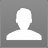Categoty :
elaine : If Arithmetic Mean and Geometric Mean of roots of a quadratic equation are 12 and 8 respectively, then identify the quadratic equation in x.: If Arithmetic Mean and Geometric Mean of roots of a quadratic equation are 12 and 8 respectively, then identify the quadratic equation in x. x2 - 24x + 64 = 0#0#x2 + 24x + 64 = 0#0#x2 - 12x + 64 = 0#0#x2 - 12x + 8 = 0#0#